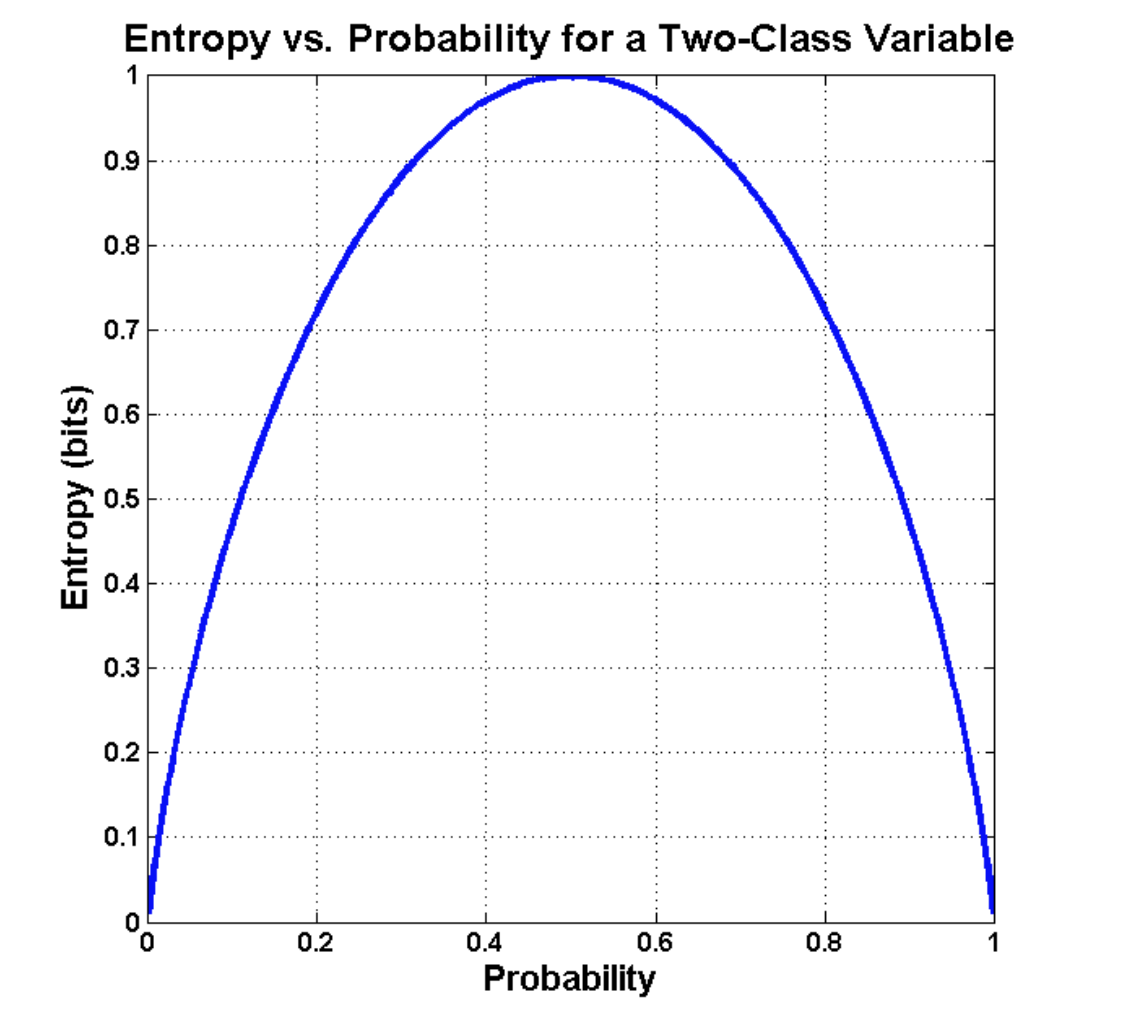# Decision Tree- An Overview

Decision Tree Split Methods

Decision tree is one of the most popular supervised machine learning algorithms used for classification as well as regression problems.

As the name suggest decision tree is a tree like model which is built upside down with its root node at the top. Root node splits into different branches, the branch end that doesn’t split anymore is the leaf node or terminal node. Each root represents a feature, each branch represents decision and each leaf represents an outcome.

Decision Tree uses layered splitting process, where at each layer layer it try to split the data into two or more groups and the data that fall into same group are most similar to each other (homogeneous) and groups are as different as possible from each other (heterogeneous).

There are different algorithms to build a decision tree but here we will discuss few of them.

• ID3 or Iterative Dichtomiser3 calculates the entropy of every attribute of the dataset, splits the dataset into subset using the attribute which has the smallest entropy or largest information gain.
• CART generates both classification and regression trees. It uses Gini index to split the node unlike ID3 which uses entropy for splitting. CART follows a greedy algorithm which aims only to reduce the cost function.

Decision tree usually consider the entire data as a root. It further splits into decision nodes/branches until leaf or terminal nodes are formed. It selects the attribute as a root node based on Gini index, entropy and Information gain.

1. ENTROPY: Entropy is the measure of impurity or uncertainty present in the dataset. It controls how a decision tree decide to split the data. Entropy ranges from 0 to 1. Entropy is nearly zero for homogeneous sample which means it is a leaf node though it can’t be divided further. If half of the sample is of positive class and half is of the negative class then entropy is one, very high.Formula to calculate entropy (left) and the graph showing entropy vs probability (right)

2. INFORMATION GAIN: By calculating entropy measure of each attribute we can calculate their information gain. Information gain measures the reduction in entropy by subtracting the weighted entropies of each branch from the original entropy.

Information Gain is calculated for each attribute in dataset. The attribute that has the largest information gain is selected to split the dataset. Information gain is precisely the measure used by ID3 algorithm.

3. GINI INDEX: Algorithm like CART uses Gini as an impurity parameter. It is a metric to measure how often a randomly chosen element would be incorrectly classified. While building a decision tree, feature which has the least value of Gini index would be preferred. Minimum value for Gini Index is 0. Gini is calculated as -

There are several advantages and disadvantages of decision trees which we should consider before building a tree model

1. Decision tree models are easy to explain and even a naïve person can understand logic by its visualization.
2. It can handle both categorical as well as numerical data and gives better accuracy than other models.
3. Decision tree is a flexible algorithm as any missing values present in a data doesn’t affect its decision.